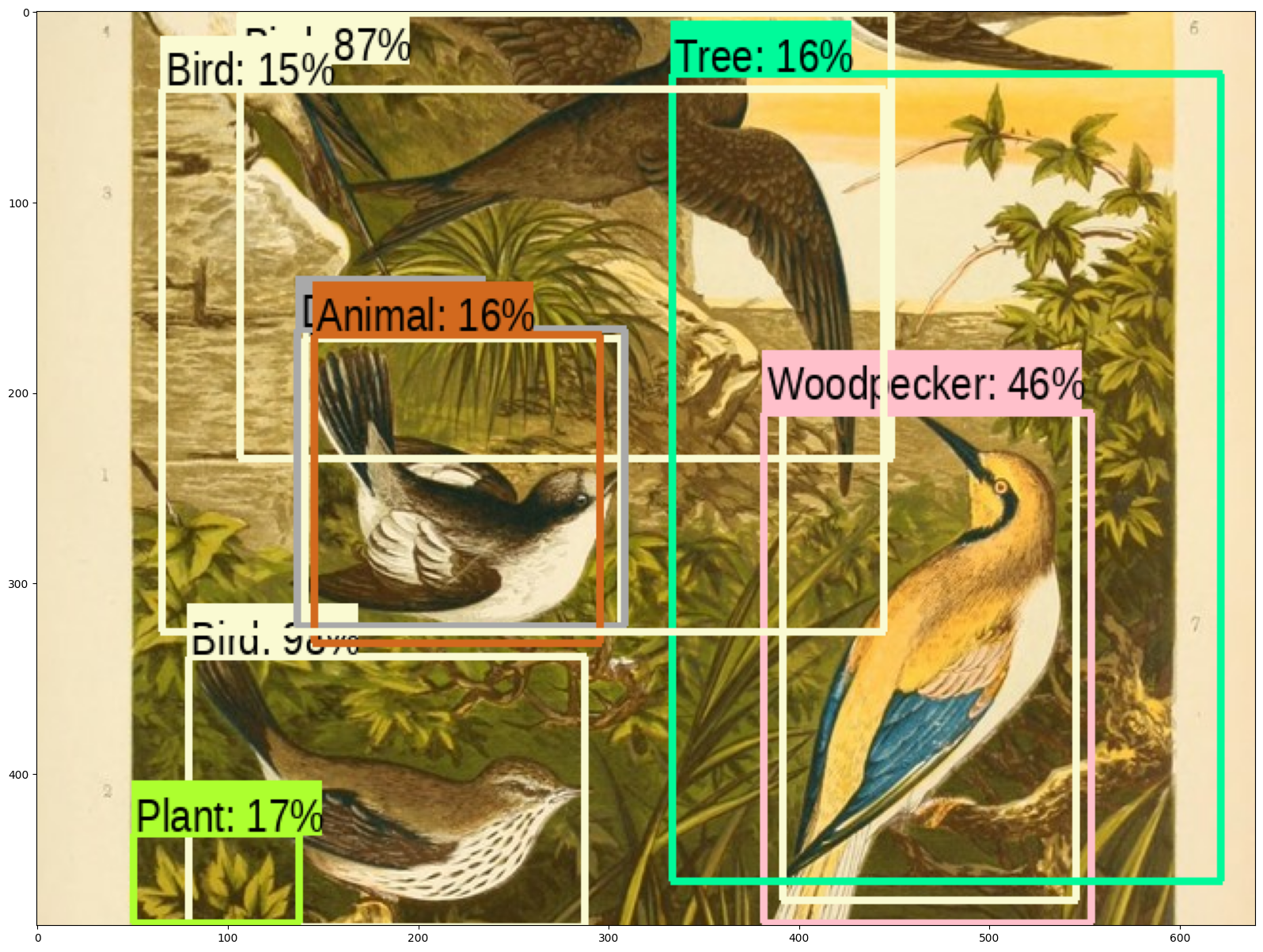Help protect the Great Barrier Reef with TensorFlow on Kaggle

对象检测

设置

Imports and function definitions

# For running inference on the TF-Hub module.
import tensorflow as tf

import tensorflow_hub as hub

import matplotlib.pyplot as plt
import tempfile
from six.moves.urllib.request import urlopen
from six import BytesIO

# For drawing onto the image.
import numpy as np
from PIL import Image
from PIL import ImageColor
from PIL import ImageDraw
from PIL import ImageFont
from PIL import ImageOps

# For measuring the inference time.
import time

# Print Tensorflow version
print(tf.__version__)

# Check available GPU devices.
print("The following GPU devices are available: %s" % tf.test.gpu_device_name())
2.6.0
The following GPU devices are available: /device:GPU:0
2021-08-13 20:24:50.878060: I tensorflow/core/platform/cpu_feature_guard.cc:142] This TensorFlow binary is optimized with oneAPI Deep Neural Network Library (oneDNN) to use the following CPU instructions in performance-critical operations:  AVX2 AVX512F FMA
To enable them in other operations, rebuild TensorFlow with the appropriate compiler flags.
2021-08-13 20:24:51.544643: I tensorflow/stream_executor/cuda/cuda_gpu_executor.cc:937] successful NUMA node read from SysFS had negative value (-1), but there must be at least one NUMA node, so returning NUMA node zero
2021-08-13 20:24:51.552971: I tensorflow/stream_executor/cuda/cuda_gpu_executor.cc:937] successful NUMA node read from SysFS had negative value (-1), but there must be at least one NUMA node, so returning NUMA node zero
2021-08-13 20:24:51.553942: I tensorflow/stream_executor/cuda/cuda_gpu_executor.cc:937] successful NUMA node read from SysFS had negative value (-1), but there must be at least one NUMA node, so returning NUMA node zero
2021-08-13 20:24:52.136749: I tensorflow/stream_executor/cuda/cuda_gpu_executor.cc:937] successful NUMA node read from SysFS had negative value (-1), but there must be at least one NUMA node, so returning NUMA node zero
2021-08-13 20:24:52.137758: I tensorflow/stream_executor/cuda/cuda_gpu_executor.cc:937] successful NUMA node read from SysFS had negative value (-1), but there must be at least one NUMA node, so returning NUMA node zero
2021-08-13 20:24:52.138637: I tensorflow/stream_executor/cuda/cuda_gpu_executor.cc:937] successful NUMA node read from SysFS had negative value (-1), but there must be at least one NUMA node, so returning NUMA node zero
2021-08-13 20:24:52.139572: I tensorflow/core/common_runtime/gpu/gpu_device.cc:1510] Created device /device:GPU:0 with 14648 MB memory:  -> device: 0, name: Tesla V100-SXM2-16GB, pci bus id: 0000:00:05.0, compute capability: 7.0

使用示例

用于下载图像和可视化的辅助函数。

def display_image(image):
fig = plt.figure(figsize=(20, 15))
plt.grid(False)
plt.imshow(image)

display=False):
_, filename = tempfile.mkstemp(suffix=".jpg")
response = urlopen(url)
image_data = BytesIO(image_data)
pil_image = Image.open(image_data)
pil_image = ImageOps.fit(pil_image, (new_width, new_height), Image.ANTIALIAS)
pil_image_rgb = pil_image.convert("RGB")
pil_image_rgb.save(filename, format="JPEG", quality=90)
if display:
display_image(pil_image)
return filename

def draw_bounding_box_on_image(image,
ymin,
xmin,
ymax,
xmax,
color,
font,
thickness=4,
display_str_list=()):
"""Adds a bounding box to an image."""
draw = ImageDraw.Draw(image)
im_width, im_height = image.size
(left, right, top, bottom) = (xmin * im_width, xmax * im_width,
ymin * im_height, ymax * im_height)
draw.line([(left, top), (left, bottom), (right, bottom), (right, top),
(left, top)],
width=thickness,
fill=color)

# If the total height of the display strings added to the top of the bounding
# box exceeds the top of the image, stack the strings below the bounding box
display_str_heights = [font.getsize(ds) for ds in display_str_list]
# Each display_str has a top and bottom margin of 0.05x.
total_display_str_height = (1 + 2 * 0.05) * sum(display_str_heights)

if top > total_display_str_height:
text_bottom = top
else:
text_bottom = top + total_display_str_height
# Reverse list and print from bottom to top.
for display_str in display_str_list[::-1]:
text_width, text_height = font.getsize(display_str)
margin = np.ceil(0.05 * text_height)
draw.rectangle([(left, text_bottom - text_height - 2 * margin),
(left + text_width, text_bottom)],
fill=color)
draw.text((left + margin, text_bottom - text_height - margin),
display_str,
fill="black",
font=font)
text_bottom -= text_height - 2 * margin

def draw_boxes(image, boxes, class_names, scores, max_boxes=10, min_score=0.1):
"""Overlay labeled boxes on an image with formatted scores and label names."""
colors = list(ImageColor.colormap.values())

try:
font = ImageFont.truetype("/usr/share/fonts/truetype/liberation/LiberationSansNarrow-Regular.ttf",
25)
except IOError:

for i in range(min(boxes.shape, max_boxes)):
if scores[i] >= min_score:
ymin, xmin, ymax, xmax = tuple(boxes[i])
display_str = "{}: {}%".format(class_names[i].decode("ascii"),
int(100 * scores[i]))
color = colors[hash(class_names[i]) % len(colors)]
image_pil = Image.fromarray(np.uint8(image)).convert("RGB")
draw_bounding_box_on_image(
image_pil,
ymin,
xmin,
ymax,
xmax,
color,
font,
display_str_list=[display_str])
np.copyto(image, np.array(image_pil))
return image

应用模块

# By Heiko Gorski, Source: https://commons.wikimedia.org/wiki/File:Naxos_Taverna.jpg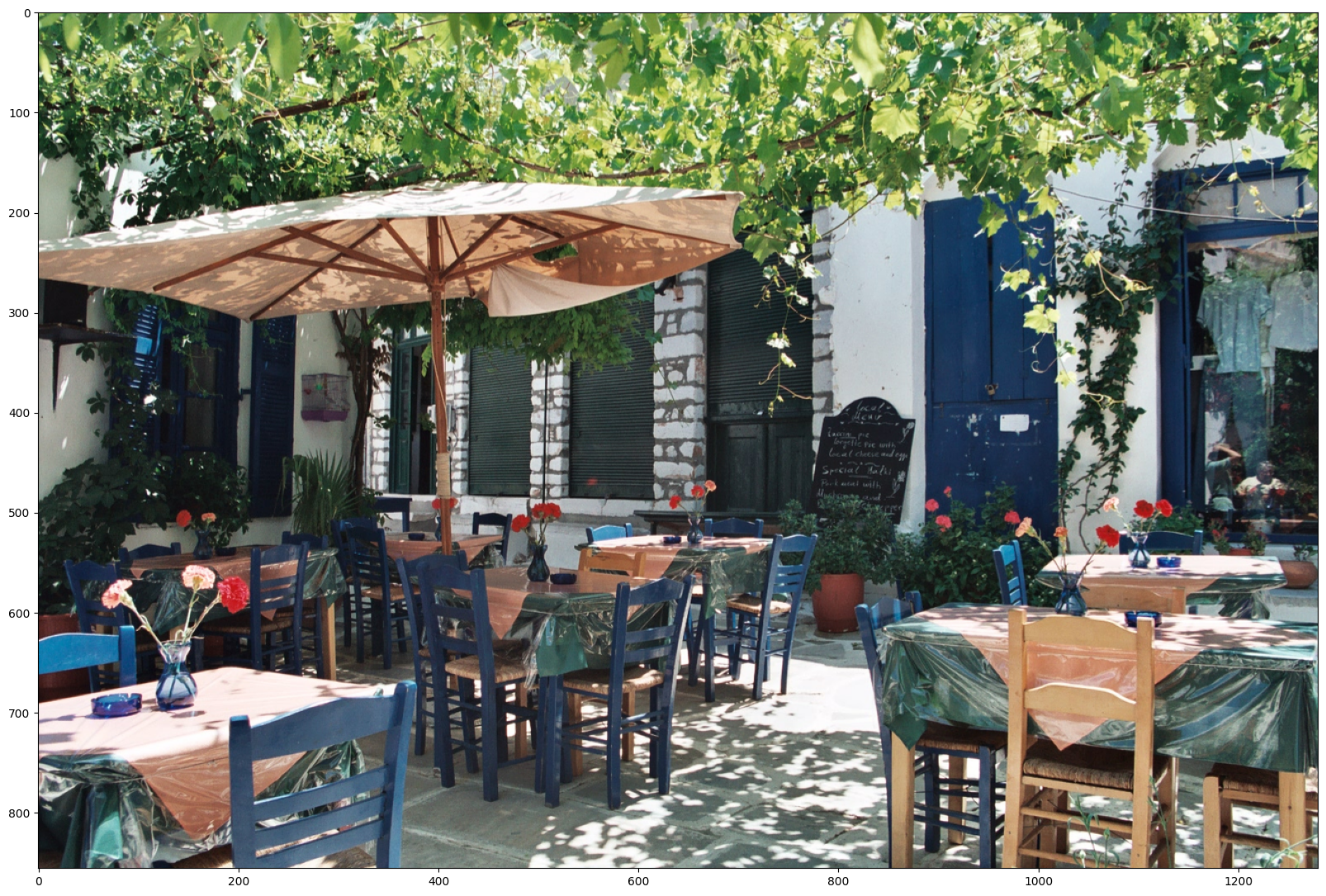• FasterRCNN+InceptionResNet V2：高准确率。
• ssd+mobilenet V2：小而快。

INFO:tensorflow:Saver not created because there are no variables in the graph to restore
INFO:tensorflow:Saver not created because there are no variables in the graph to restore
2021-08-13 20:25:19.500739: I tensorflow/stream_executor/cuda/cuda_gpu_executor.cc:937] successful NUMA node read from SysFS had negative value (-1), but there must be at least one NUMA node, so returning NUMA node zero
2021-08-13 20:25:19.501860: I tensorflow/stream_executor/cuda/cuda_gpu_executor.cc:937] successful NUMA node read from SysFS had negative value (-1), but there must be at least one NUMA node, so returning NUMA node zero
2021-08-13 20:25:19.502734: I tensorflow/stream_executor/cuda/cuda_gpu_executor.cc:937] successful NUMA node read from SysFS had negative value (-1), but there must be at least one NUMA node, so returning NUMA node zero
2021-08-13 20:25:19.503916: I tensorflow/stream_executor/cuda/cuda_gpu_executor.cc:937] successful NUMA node read from SysFS had negative value (-1), but there must be at least one NUMA node, so returning NUMA node zero
2021-08-13 20:25:19.504789: I tensorflow/stream_executor/cuda/cuda_gpu_executor.cc:937] successful NUMA node read from SysFS had negative value (-1), but there must be at least one NUMA node, so returning NUMA node zero
2021-08-13 20:25:19.505612: I tensorflow/stream_executor/cuda/cuda_gpu_executor.cc:937] successful NUMA node read from SysFS had negative value (-1), but there must be at least one NUMA node, so returning NUMA node zero
2021-08-13 20:25:19.506504: I tensorflow/stream_executor/cuda/cuda_gpu_executor.cc:937] successful NUMA node read from SysFS had negative value (-1), but there must be at least one NUMA node, so returning NUMA node zero
2021-08-13 20:25:19.507333: I tensorflow/stream_executor/cuda/cuda_gpu_executor.cc:937] successful NUMA node read from SysFS had negative value (-1), but there must be at least one NUMA node, so returning NUMA node zero
2021-08-13 20:25:19.508120: I tensorflow/core/common_runtime/gpu/gpu_device.cc:1510] Created device /job:localhost/replica:0/task:0/device:GPU:0 with 14648 MB memory:  -> device: 0, name: Tesla V100-SXM2-16GB, pci bus id: 0000:00:05.0, compute capability: 7.0
2021-08-13 20:25:19.765939: I tensorflow/compiler/mlir/mlir_graph_optimization_pass.cc:185] None of the MLIR Optimization Passes are enabled (registered 2)
img = tf.image.decode_jpeg(img, channels=3)
return img
def run_detector(detector, path):

converted_img  = tf.image.convert_image_dtype(img, tf.float32)[tf.newaxis, ...]
start_time = time.time()
result = detector(converted_img)
end_time = time.time()

result = {key:value.numpy() for key,value in result.items()}

print("Found %d objects." % len(result["detection_scores"]))
print("Inference time: ", end_time-start_time)

image_with_boxes = draw_boxes(
img.numpy(), result["detection_boxes"],
result["detection_class_entities"], result["detection_scores"])

display_image(image_with_boxes)
2021-08-13 20:26:36.858585: I tensorflow/stream_executor/cuda/cuda_dnn.cc:369] Loaded cuDNN version 8100
2021-08-13 20:26:37.404006: I tensorflow/core/platform/default/subprocess.cc:304] Start cannot spawn child process: No such file or directory
Found 100 objects.
Inference time:  33.998905420303345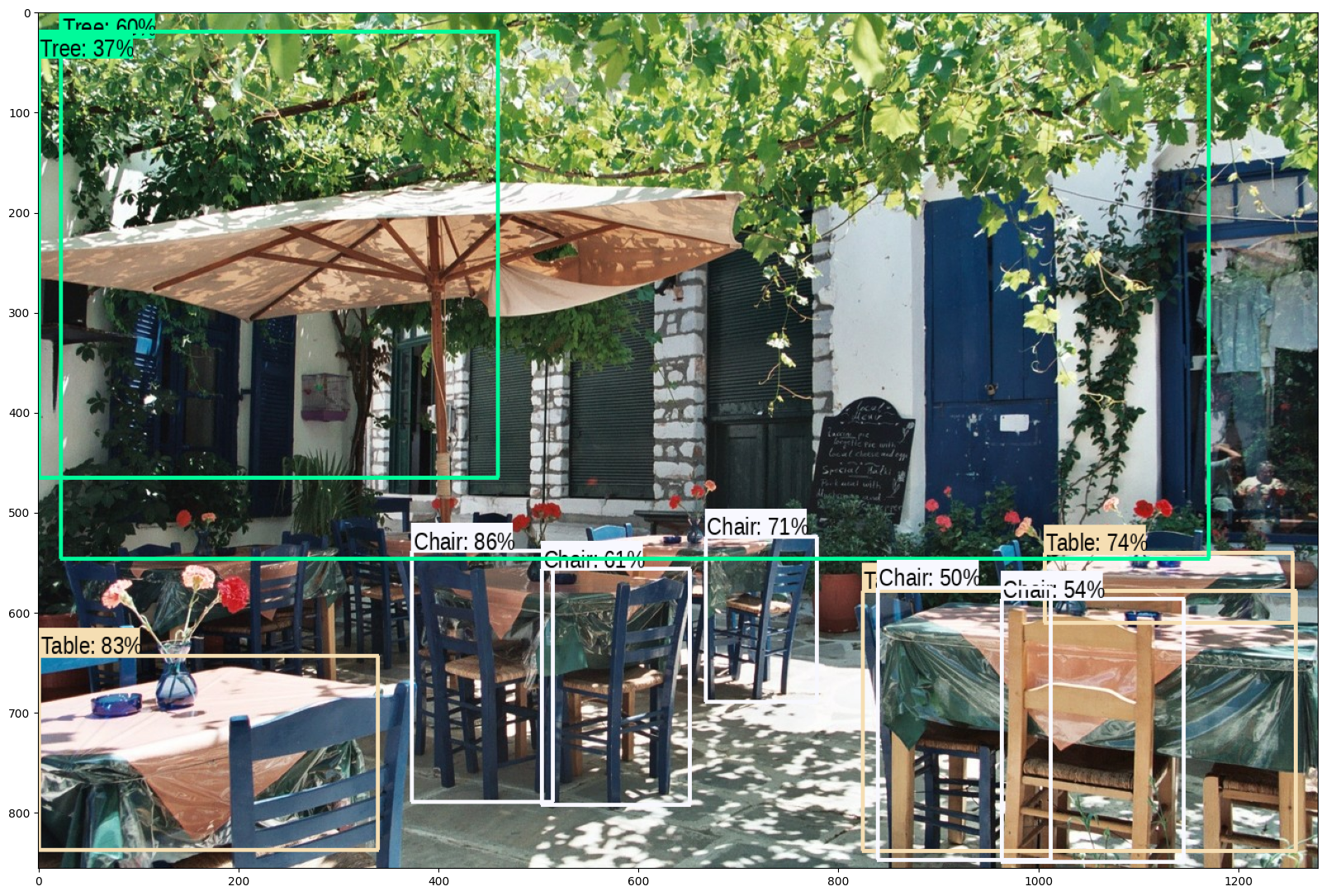更多图像

image_urls = [
# Source: https://commons.wikimedia.org/wiki/File:The_Coleoptera_of_the_British_islands_(Plate_125)_(8592917784).jpg
# By Américo Toledano, Source: https://commons.wikimedia.org/wiki/File:Biblioteca_Maim%C3%B3nides,_Campus_Universitario_de_Rabanales_007.jpg
# Source: https://commons.wikimedia.org/wiki/File:The_smaller_British_birds_(8053836633).jpg
]

def detect_img(image_url):
start_time = time.time()
run_detector(detector, image_path)
end_time = time.time()
print("Inference time:",end_time-start_time)
detect_img(image_urls)
Found 100 objects.
Inference time:  1.394282579421997
Inference time: 2.003801107406616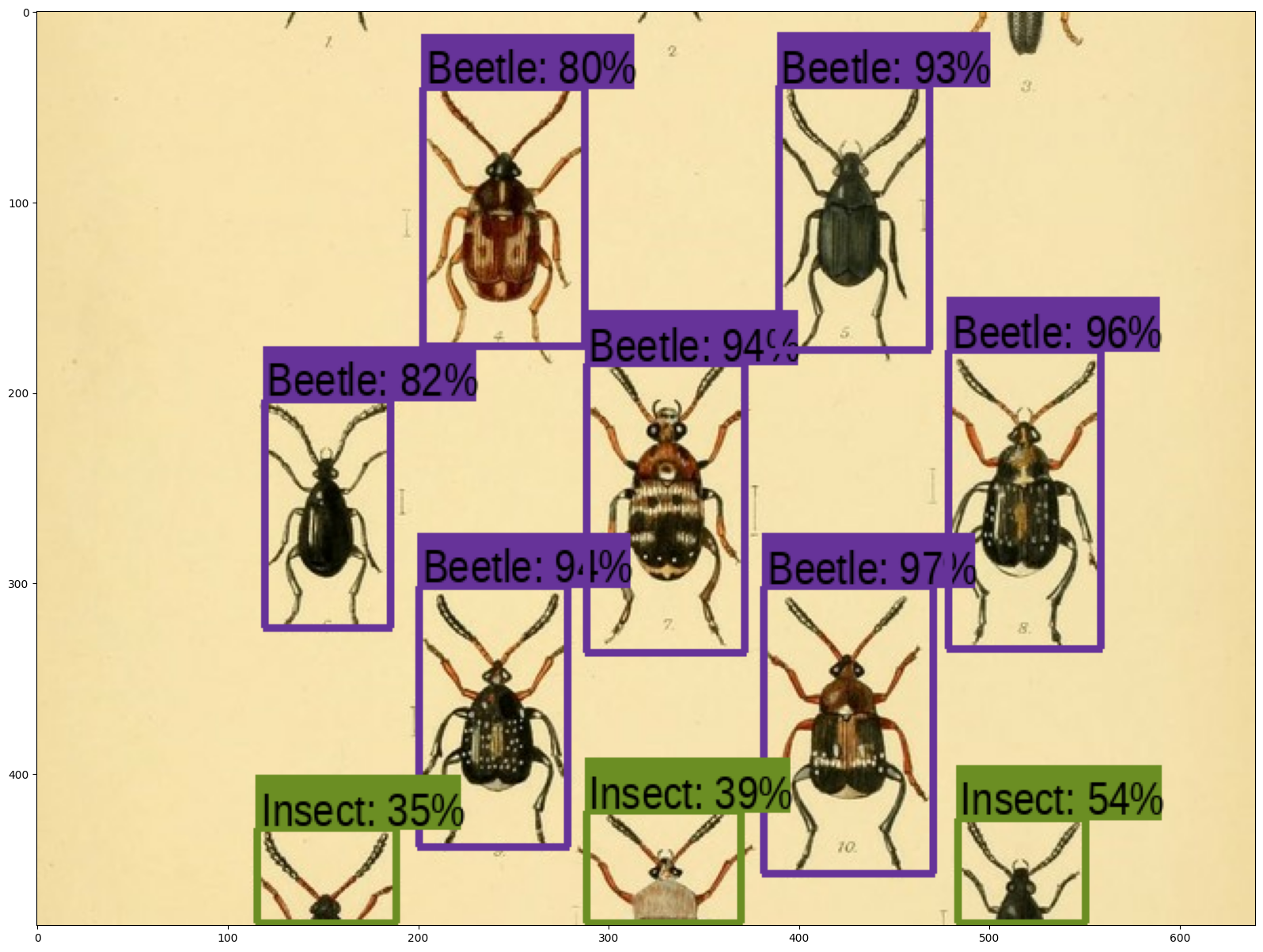detect_img(image_urls)
Found 100 objects.
Inference time:  0.8170506954193115
Inference time: 1.2012197971343994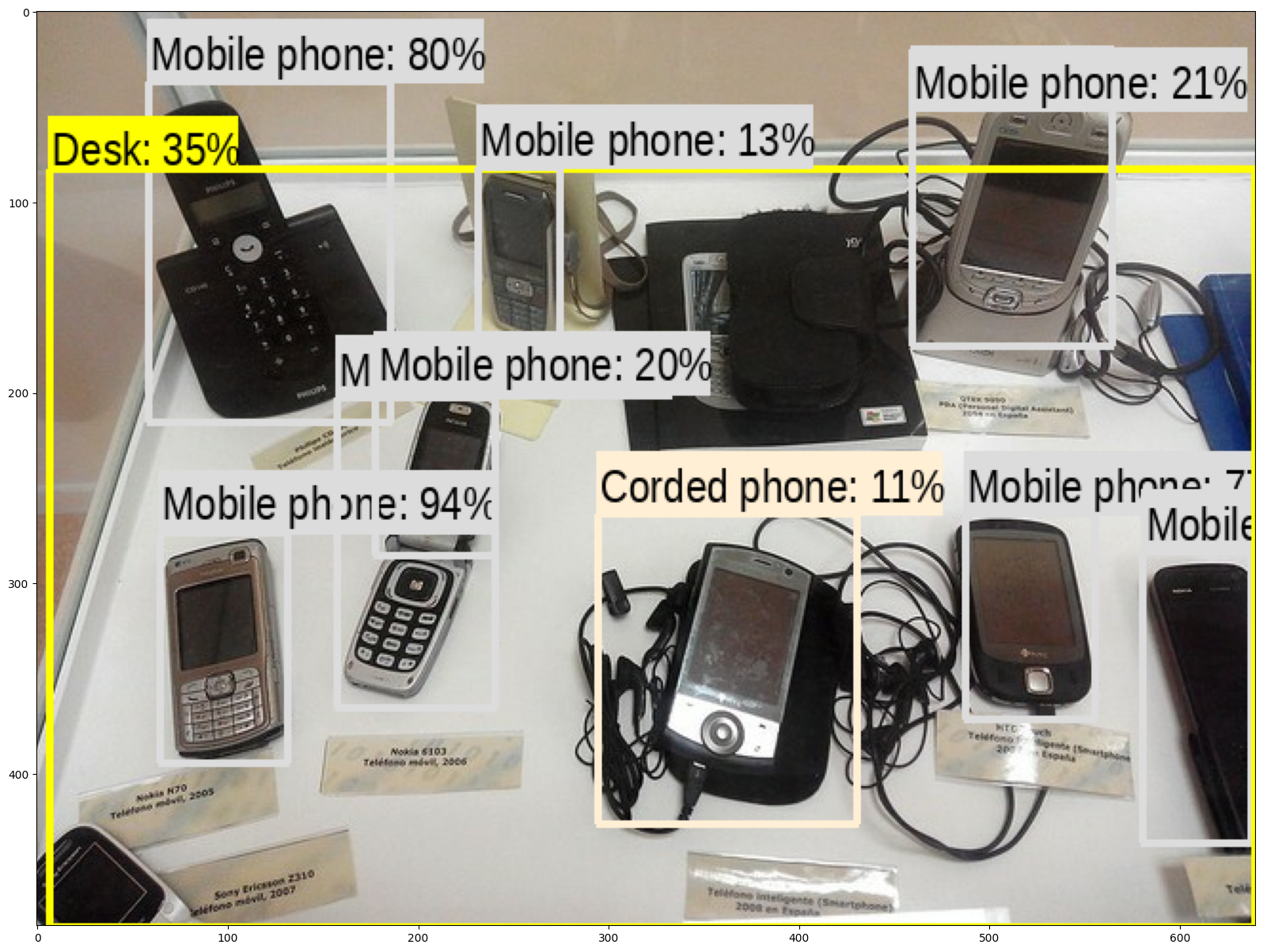detect_img(image_urls)